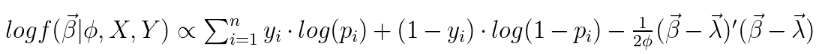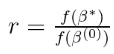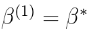# 模型

1. 我们有一个二进制结果，为此我们采用了非线性链接函数。
2. 我们有一个需要调整的因素。
3. 我们正在估计我们关心的更多参数，但肯定会给采样器带来压力。

# 非规范条件后验# Metroplis-in-Gibbs采样

MH采样器的工作方式如下：

R语言中RStan贝叶斯层次模型分析示例

1. 开始采样。
2. 让我们假设将提议分布的方差设置为某个常数。
3. 我们计算在上一次绘制时评估的非标准化密度与当前提议的比率：4. 如果该比率大于1，则当前提议分布的密度高于先前值的密度。因此，我们“接受”了提议并确定了。然后，我们使用以提议为中心的提议分布重复步骤2-4  ，然后生成新提议。如果该比率小于1，则当前建议值的密度低于先前建议。

• 跳跃分布的方差是重要的参数。如果方差太小，则当前提议可能会非常接近最后一个值，因此也很可能接近1。因此，我们会非常频繁地接受，但由于接受的值彼此之间非常接近，因此我们会攀升至较高在许多次迭代中慢慢降低密度区域。如果方差太大，则序列到达高密度区域后可能无法保留在该区域。
• 许多“自适应” MH方法是此处描述的基本算法的变体，但包括调整周期以找到产生最佳接受率的跳跃分布方差。
• MH中计算量最大的部分是密度评估。对于每个Gibbs迭代，我们必须两次评估4维密度。
• 尽管很容易扩展到高维度，但性能本身在高维度上会变差。

# 结果

``````profvis(expr = {
for(i in 2:gibbs_iter){
# 来自 phi 后验分布的样本
gibbs_res[i,p+1] <- rcond_post_phi(gibbs_res[i-1,1:p],
alpha, gamma, lambda, p)
#  来自beta后验分布的样本 （ 使用 Mh ）
mh_draw <- rcond_post_beta_mh(gibbs_res[i-1,1:p], gibbs_res[i,p+1],
lambda, X, Y, mh_trials=5, jump_v=.01)
}
})
par(mfrow=c(2,2))
plot(gibbs_res[,1],type='l',xlab='MCMC Iterations',ylab=c('Coefficient Draw'),
main='Intercept')
abline(h=-1,col='red')
plot(gibbs_res[,2],type='l',xlab='MCMC Iterations',ylab=c('Coefficient Draw'),
main='Age1')
abline(h=.7,col='red')
plot(gibbs_res[,3],type='l',xlab='MCMC Iterations',ylab=c('Coefficient Draw'),
main='Age2')
abline(h=1.1,col='red')
plot(gibbs_res[,4],type='l',xlab='MCMC Iterations',ylab=c('Coefficient Draw'),
main='Treatment')
abline(h=1.1,col='red')
# 计算后验分布和置信区间
post_burn_trim<-gibbs_res[seq(1000,gibbs_iter,100),]
colMeans(post_burn_trim)
apply(post_burn_trim, 2, quantile, p=c(.025,.975))``````

• 接受率只有18％，我本可以调整跳跃分布协方差矩阵来获得更好的接受率。
• 我认为更多的迭代肯定会在这里有所帮助。这些链看起来不错，但仍然是自相关的。

# 关于贝叶斯范式的好处是，所有推断都是使用后验分布完成的。现在，系数估计值是对数化，但是如果我们需要比值，则只需对后验取幂。

### 随时关注您喜欢的主题

Kaizong Ye拓端研究室（TRL）的研究员。

​非常感谢您阅读本文，如需帮助请联系我们！QQ在线咨询

15121130882

0571-63341498

## 关注有关新文章的微信公众号

This will close in 0 seconds